# 【深度学习】新的深度学习优化器探索（协同优化）1人已赏
Fizz 发布于 2021-06-17 17:33:50 浏览 2617 点赞 96 收藏 5

## 【深度学习】新的深度学习优化器探索（协同优化）``````文章目录
2 自适应优化
3.1 “侵入式”优化器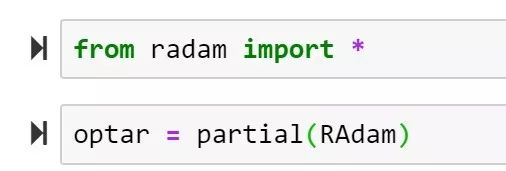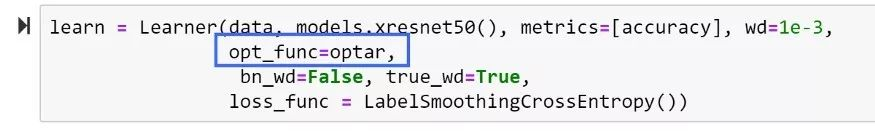# 2 自适应优化

Moment Estimation，即梯度的未中心化的方差）进行综合考虑，计算出更新步长。

1. 实现简单，计算高效，对内存需求少

2. 参数的更新不受梯度的伸缩变换影响

3. 超参数具有很好的解释性，且通常无需调整或仅需很少的微调

4. 更新的步长能够被限制在大致的范围内（初始学习率）

5. 能自然地实现步长退火过程（自动调整学习率）

6. 很适合应用于大规模的数据及参数的场景

7. 适用于不稳定目标函数

8. 适用于梯度稀疏或梯度存在很大噪声的问题

``x += - learning_rate * dx``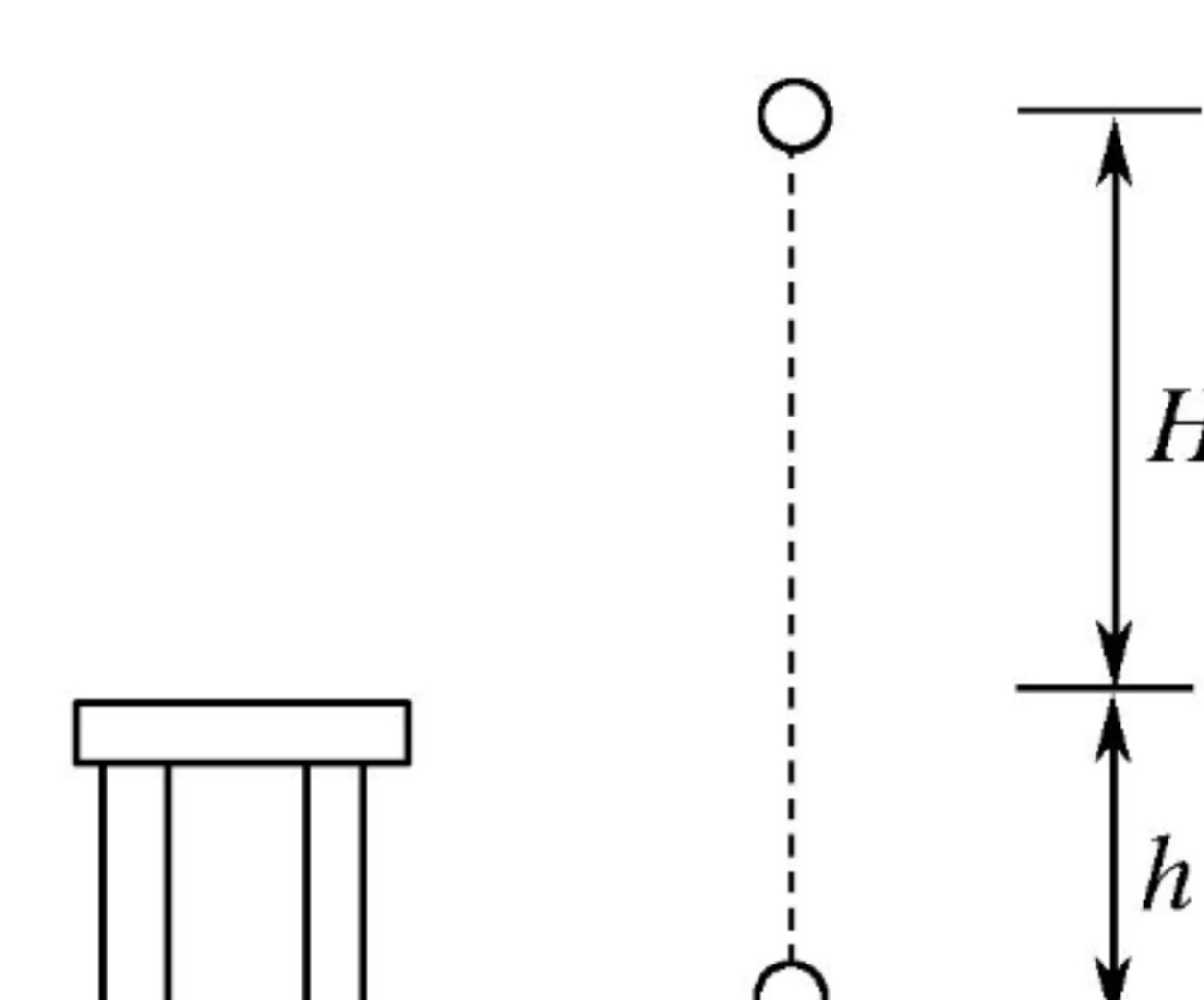``````v = mu * v - learning_rate * dx # 与速度融合
x += v # 与位置融合``````

``````1、备份模型现有的权重θ；

2、从θ出发，用指定优化器更新k步，得到新权重θ̃ ；

3、更新模型权重为θ←θ+α(θ̃ −θ)。``````

## 3.1 “侵入式”优化器

``````from keras.optimizers import Optimizer
from keras import backend as K

class InjectOptimizer(Optimizer):
"""定义注入式优化器的基类
需要传入模型，直接修改模型的训练函数，而不按常规流程使用优化器，所以称为“侵入式”
其实下面的大部分代码，都是直接抄自keras的源码：
https://github.com/keras-team/keras/blob/master/keras/engine/training.py#L497
也就是keras中的_make_train_function函数。
"""
return []
raise NotImplementedError
def inject(self, model):
"""传入模型做注入
"""
if not hasattr(model, 'train_function'):
raise RuntimeError('You must compile your model before using it.')
model._check_trainable_weights_consistency()
if model.train_function is None:
inputs = (model._feed_inputs +
model._feed_targets +
model._feed_sample_weights)
if model._uses_dynamic_learning_phase():
inputs += [K.learning_phase()]
with K.name_scope('training'):
train_functions = []
with K.name_scope(model.optimizer.__class__.__name__):
params=model._collected_trainable_weights,
loss=model.total_loss)
f = K.function(
inputs,
[model.total_loss],
name='train_function_%s' % (i + 1),
**model._function_kwargs)
train_functions.append(f)
# Gets loss and metrics. Updates weights at each call.
first_train = K.function(
inputs,
[model.total_loss] + model.metrics_tensors,
name='train_function',
**model._function_kwargs)
def F(inputs):
R = first_train(inputs)
for f in train_functions:
f(inputs)
return R
model.train_function = F

class HeunOptimizer(InjectOptimizer):
"""Heun优化器
( https://en.wikipedia.org/wiki/Heun%27s_method )
"""
def __init__(self, lr, **kwargs):
super(HeunOptimizer, self).__init__(**kwargs)
with K.name_scope(self.__class__.__name__):
self.lr = K.variable(lr, name='lr')
updates.append(K.update(p, p - self.lr * g))
updates.append(K.update(p, p - 0.5 * self.lr * (g - cg)))
cache_grads = [K.zeros(K.int_shape(p)) for p in params]
return [
]
``````

``````opt = HeunOptimizer(0.1)
model.compile(loss='mse', optimizer=opt)
opt.inject(model) # 必须执行这步

model.fit(x_train, y_train, epochs=100, batch_size=32)``````

``````model.compile(optimizer=Adam(1e-3), loss='mse') # 用你想用的优化器

Lookahead 迭代地更新两组权重：slow weights φ 和 fast weights θ，前者在后者每更新 k 次后更新一次。Lookahead 将任意标准优化算法 A 作为内部优化器来更新 fast weights。

slow weights 每更新一次，fast weights 将被重置为目前的 slow weights 值。Lookahead 的伪代码见下图 Algorithm 1。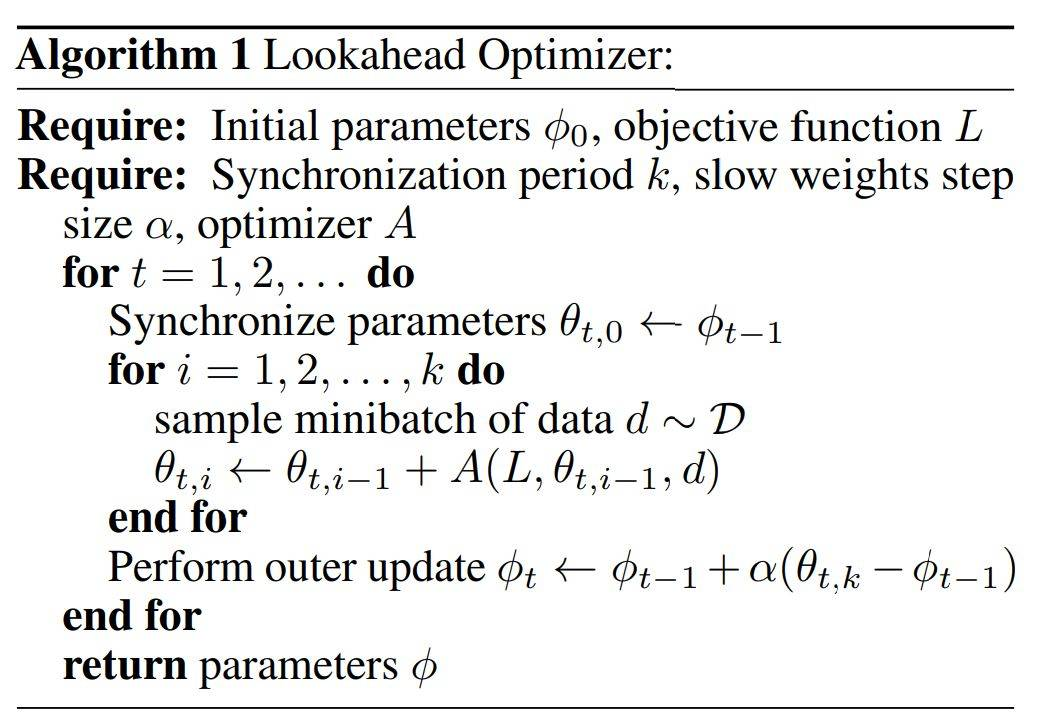其中最优化器 A 可能是 Adam 或 SGD 等最优化器，内部的 for 循环会用常规方法更新 fast weights θ，且每次更新的起始点都是从当前的 slow weights φ 开始。最终模型使用的参数也是慢更新那一套，因此快更新相当于做了一系列实验，然后慢更新再根据实验结果选一个比较好的方向，这有点类似 Nesterov Momentum 的思想。

k - 它控制快优化器的权重和 LookAhead 中的慢优化器的权重协同更新的间隔。默认值一般是 5 或者 6，不过 LookAhead 论文里最大也用过 20。 alpha - 它控制根据快慢优化器权重之差的多少比例来更新快优化器的权重。默认值是 0.5，LookAhead 论文作者 Hinton 等人在论文里给出了一个强有力的证明，表示 0.5 可能就是理想值。不过大家也可以做自己的尝试。 他们也在论文中指出，未来一个可能的改进方向是根据训练进行到不同的阶段，规划使用不同的 k 和 alpha 的值。

Ranger 的代码开源在 https://github.com/lessw2020/Ranger-Deep-Learning-Optimizer?source=post_page-----2dc83f79a48d----------------------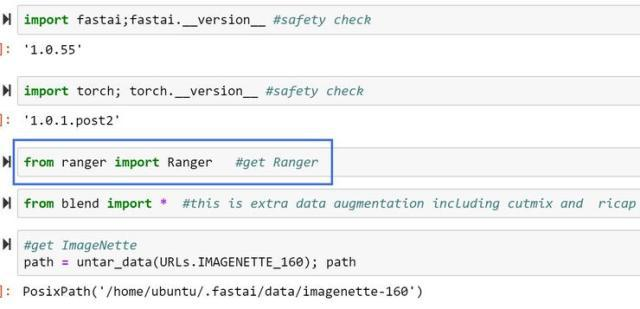*本文仅代表作者观点，不代表易百纳技术社区立场。系作者授权易百纳技术社区发表，未经许可不得转载。

### 相关专栏海思讨论群君正智能视频群客服微信： x460910098客服手机： 17714209983